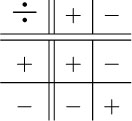Science, Maths & Technology

### Become an OU studentNumbers, units and arithmetic

Start this free course now. Just create an account and sign in. Enrol and complete the course for a free statement of participation or digital badge if available.

# 3.21 Division rules

Lastly consider division. Dividing 8 by 2 means ‘How many times does 2 go into 8?’ or ‘What must you multiply 2 by to get 8?’. The answer is 4.

So to find 8 ÷ 2, you need to ask ‘What do I have to multiply 2 by to get 8?’. The answer is 4, since 2 × 4 = 8.

So 8 ÷ 2 = 4.

Similarly, to find 8 ÷ 2 you need to ask ‘what do I have to multiply 2 by to get 8?’ and the answer is 4, since 2 × 4 = 8.

So 8 ÷ 2 = 4.

Using this sort of argument you can work out the rules for division of and by negative numbers. They are remarkably similar to those for multiplication (as you might expect, since they are inverse processes).

Multiplying or dividing a positive number by a positive number gives a positive answer.

Multiplying or dividing a negative number by a positive number gives a negative answer.

Multiplying or dividing a positive number by a negative number gives a negative answer.

Multiplying or dividing a negative number by a negative number gives a positive answer.The last one is the most difficult to remember. If you are in doubt, you can always use your calculator to check.

These rules may be summarised as follows.

Multiplying or dividing two numbers of the same sign gives a positive answer.

Multiplying or dividing two numbers of different signs gives a negative answer.

## Try some yourself

### Activity 62

Give examples of all the rules for the division of and by positive and negative numbers.

Your list should look something like this.

Dividing a positive number by a positive number gives a positive answer.

4 ÷ 2 = 2.

Dividing a negative number by a positive number gives a negative answer.

4 ÷ 2 = 2.

Dividing a positive number by a negative number gives a negative answer.

4 ÷ 2 = 2.

Dividing a negative number by a negative number gives a positive answer.

4 ÷ 2 = 2.

### Activity 63

Evaluate each of the following and where possible give an example from everyday life to illustrate the calculation.

• (a) 24 ÷ 4

• (b) 40 ÷ 4

• (c) 45 ÷ 15

• (a) 24 ÷ 4 = 6

• Thomas has lots of £4 IOUs. A gift of £24 will subtract 6 of them.

• (b) 40 ÷ 4 = 10

• An IOU of £40 divided into smaller IOUs of £4 each (which could be paid off more easily from Thomas's pocket money) gives 10 smaller IOUs.

• (c) 45 ÷ 15 = 3

• A debt of £45 shared between 15 people means each owes £3.

### Activity 64

Evaluate each of the following.

• (a) 7 × 6

• (b) 56 ÷ 7

Think of a financial context where each might be an appropriate calculation (bear in mind that negative numbers can represent debts).